## machine learning 之 Neural Network 2

• Neural network and classification
• Cost function
• Backpropagation （to minimize cost function）
• Backpropagation in practice
• Random initialization
• Assure structure and Train a neural network

L：神经网络的总的层数，total layers，如下图，L=4

$s_l$：第l层的单元数目，如下图，$s_1=3, s_2=5,...$

K：输出层的单元数，如下图，K=4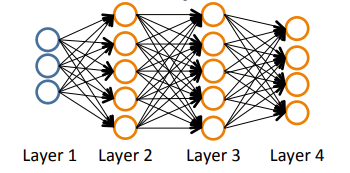1、neural network and classification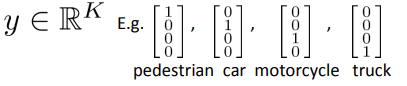2、cost function

$J(\theta)=-\frac{1}{m}[\sum_{i=1}^m y^{(i)}logh_\theta(x^{(i)}) + (1-y^{(i)})log(1-h_\theta(x{(i)}))] + \frac{\lambda}{2m} \sum_{j=1}^n \theta_j^2$

more generally，在神经网络中，$h_\Theta(x) \in R^K，(h_\Theta(x))_i是第i个output$，神经网络的cost function为：

$J(\Theta)=-\frac{1}{m}[\sum_{i=1}^m \sum_{k=1}^K y_k^{(i)}log(h_\Theta(x^{(i)})_k) + (1-y_k^{(i)})log(1-(h_\Theta(x{(i)}))_k)] + \frac{\lambda}{2m} \sum_{l=1}^{L-1} \sum_{i=1}^{s_l} \sum_{j=1}^{s_{l+1}} (\Theta_{ji}^{(l)})^2$

• 需要把K个输出的损失相加，所以有了K个累加项；
• 在惩罚项里面，需要把除bias unit以外的所有的参数（除$\Theta_0以外的所有\Theta$）都进行惩罚；

3、Backpropagation

• 首先进行forward propagation，计算出每一层的单元值（包括输出层的值）；（上一篇文章的内容）
• 进行backpropagation：（以前提中的神经网络为例）
• 设定$\delta_k^{(l)}$：为第l层上第j个结点的误差error，那么$\delta_j^{(4)}=a_j^{(4)}-y_j$，给出 $\delta^{(l)}=(\theta^{(l)})^T\delta^{(l+1)}.*g^{'}(z^{(l)})$，其中$g^{'}(z^{(l)})=a^{(l)}.*(1-a^{(l)})$

• 注意：没有$\delta^{(1)}$，因为$\delta^{(1)}$是观测数据，不存在误差一说，由于在此处计算误差是从后往前，所以这个算法被称为backpropagation
• 给出公式 $\frac{\partial J(\theta)}{\partial \theta_{ij}^{(l)}}=a_j^{(l)}\delta_i^{(l+1)}$
• 对于每一个训练数据，计算它们的偏导数，并且将其相加，$\Delta^{(l)}:=\Delta^{(l)}+\delta^{(l+1)}(a^{(l)})^T$
• 在所有的训练数据处理完之后，计算损失函数对每一个参数的偏导数，也就是参数的更新参数，$D_{ij}^{(l)}=\frac{1}{m}\Delta_{ij}^{(l)}+\lambda\Theta_{ij}^{(l)}$，当j为0时，$\lambda$为0（$\theta_0$不惩罚）

4、Backpropagation in practice

unrolling parameters：把矩阵形式的参数展开成向量，为了使用已有的函数对损失函数进行最小化运算；（matrix to vector）

reshape：vector to matrix，在计算偏导数和损失函数时，矩阵运算

做了backpropagation计算了损失函数对每个参数的偏导数之后，我们需要做一个checking来确定偏导数的计算是否正确，数值计算梯度如图所示：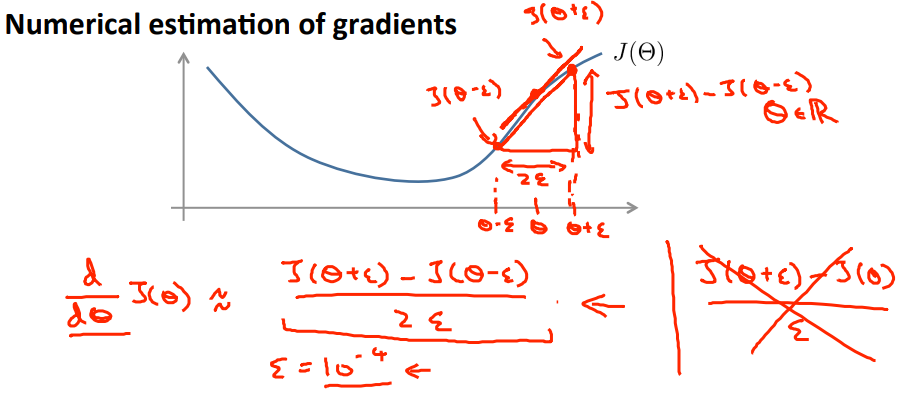$\frac{J(\theta+\varepsilon)-J(\theta-\varepsilon)}{2\varepsilon}$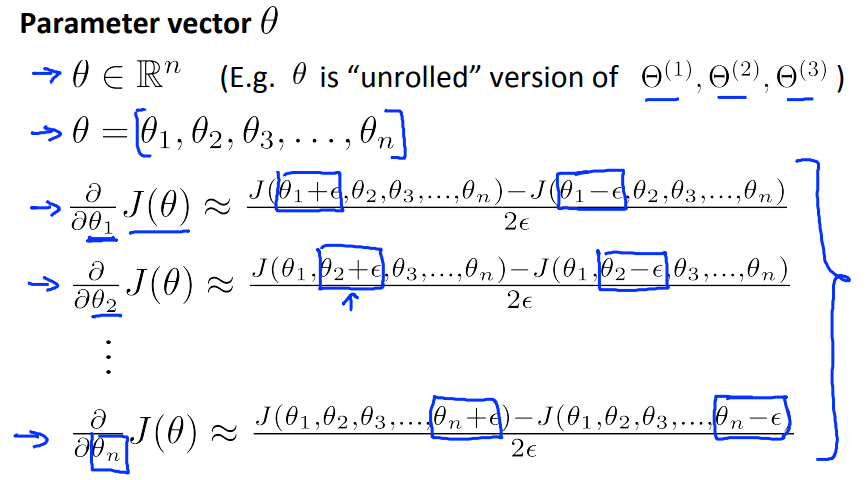6、Random initalization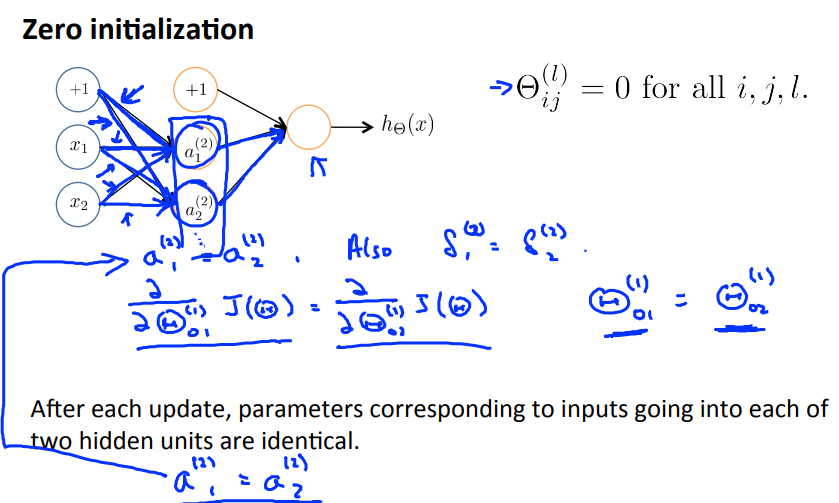7、Assure structure and Train a neural network

• 输入单元数：$x^{(i)}$的维度（$x^{(i)}$代表第i个训练数据）
• 输出单元数：类别数
• 每一个隐含层的单元数：通常多多益善
• 默认一个hidden layer，如超过了一个hidden layer，那就默认每一层的单元数相同

1. 随机初始化权重参数$\Theta$
2. 使用forward propagation计算每一层的单元的值（包括输出层的值）
3. 根据以上公式计算cost function
4. 使用backpropagation计算偏导数
6. 使用gradient descent或者其他的优化函数去最小化cost function，得到权重参数$\Theta$